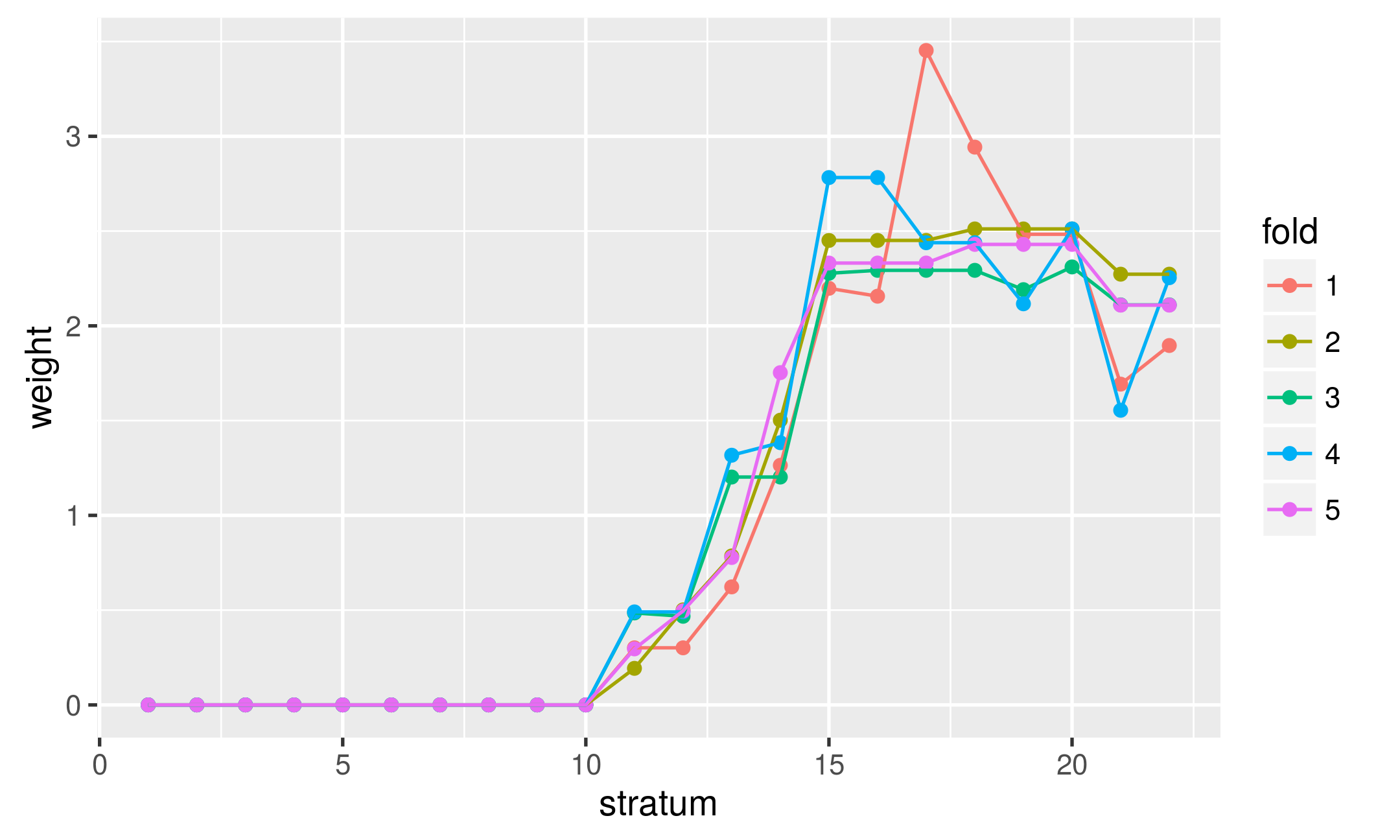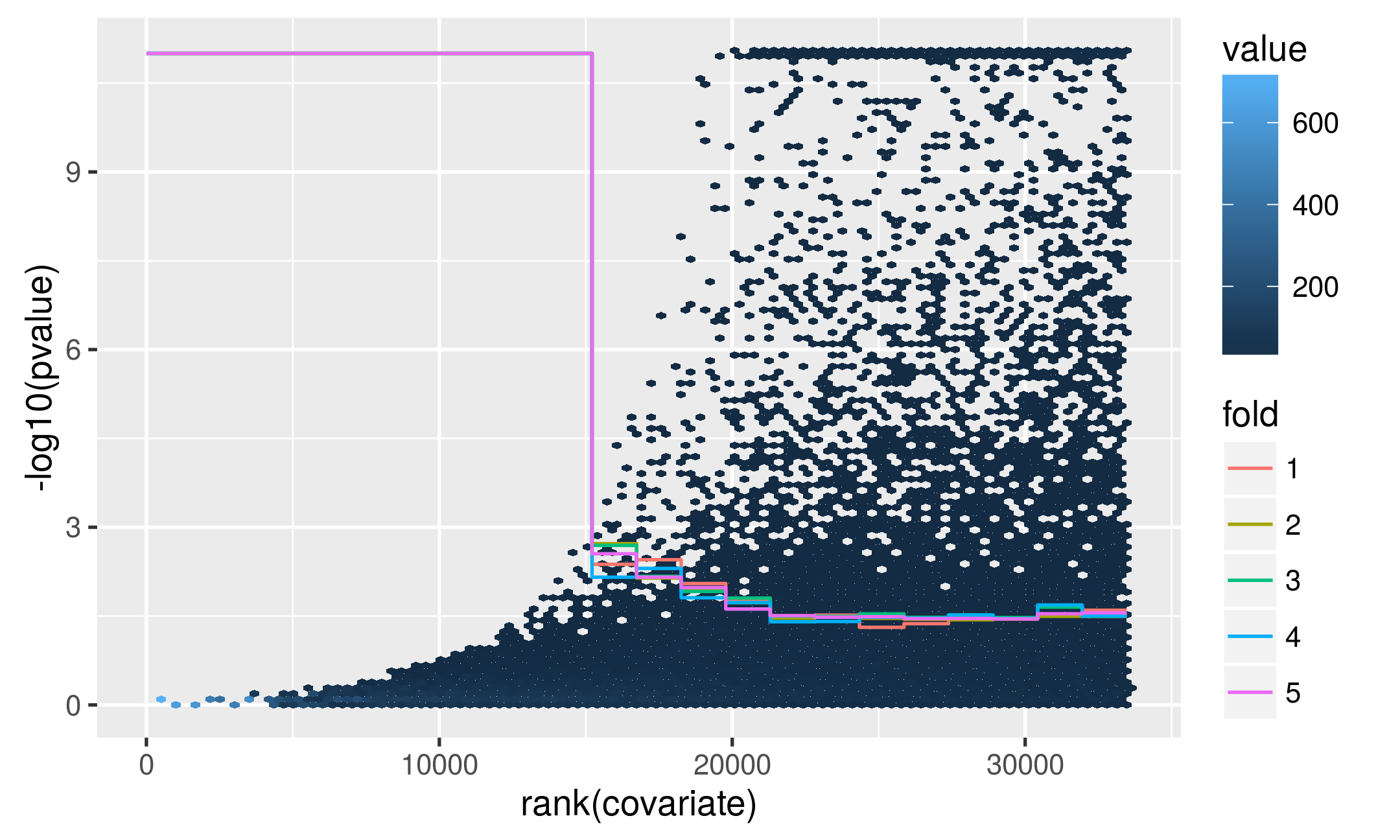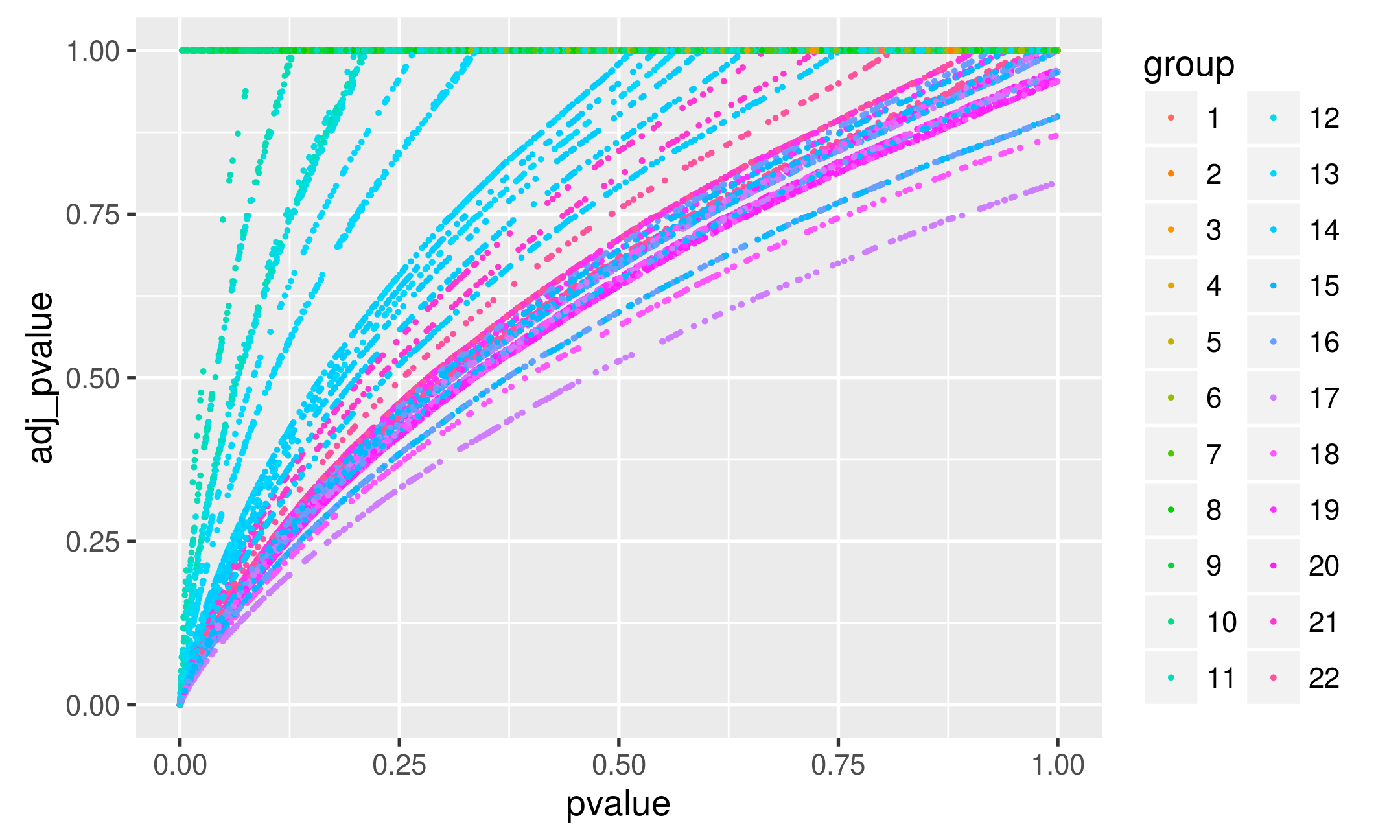# 1 Introduction

You will probably be familiar with multiple testing procedures that take a set of p-values and then calculate adjusted p-values. Given a significance level $$\alpha$$, one can then declare the rejected hypotheses. In R this is most commonly done with the p.adjust function in the stats package, and a popular choice is controlling the false discovery rate (FDR) with the method of (Benjamini and Hochberg 1995), provided by the choice method="BH" in p.adjust. A characteristic feature of this and other methods –responsible both for their versatility and limitations– is that they do not use anything else beyond the p-values: no other potential information that might set the tests apart, such as different signal quality, power, prior probability.

IHW (Independent Hypothesis Weighting) is also a multiple testing procedure, but in addition to the p-values it allows you to specify a covariate for each test. The covariate should be informative of the power or prior probability of each individual test, but is chosen such that the p-values for those hypotheses that are truly null do not depend on the covariate (Ignatiadis et al. 2016). Therefore the input of IHW is the following:

• a vector of p-values (of length $$m$$),
• a matching vector of covariates,
• the significance level $$\alpha \in (0,1)$$ at which the FDR should be controlled.

IHW then calculates weights for each p-value (non-negative numbers $$w_i \geq 0$$ such that they average to 1, $$\sum_{i=1}^m w_i = m$$). IHW also returns a vector of adjusted p-values by applying the procedure of Benjamini Hochberg (BH) to the weighted p-values $$P^\text{weighted}_i = \frac{P_i}{w_i}$$.

The weights allow different prioritization of the individual hypotheses, based on their covariate. This means that the ranking of hypotheses with p-value weighting is in general different than without. Two hypotheses with the same p-value can have different weighted p-values: the one with the higher weight will then have a smaller value of $$P^\text{weighted}_i$$, and consequently it can even happen that one but not the other gets rejected by the subsequent BH procedure.

As an example, let’s see how to use the IHW package in analysing for RNA-Seq differential gene expression. and then also look at some other examples where the method is applicable.

# 2 An example: RNA-Seq differential expression

We analyze the airway RNA-Seq dataset using DESeq2 (Love, Huber, and Anders 2014).

library("DESeq2")
library("dplyr")
data("airway", package = "airway")
dds <- DESeqDataSet(se = airway, design = ~ cell + dex) %>% DESeq
deRes <- as.data.frame(results(dds))

The output is a dataframe with the following columns, and one row for each tested hypothesis (i.e., for each gene):

colnames(deRes)
##  "baseMean"       "log2FoldChange" "lfcSE"          "stat"
##  "pvalue"         "padj"

In particular, we have p-values and baseMean (i.e., the mean of normalized counts) for each gene. As argued in the DESeq2 paper, these two statistics are approximately independent under the null hypothesis. Thus we have all the ingredient necessary for a IHW analysis (p-values and covariates), which we will apply at a significance level 0.1.

## 2.1 FDR control

library("IHW")
ihwRes <- ihw(pvalue ~ baseMean,  data = deRes, alpha = 0.1)

This returns an object of the class ihwResult. We can get, e.g., the total number of rejections.

rejections(ihwRes)
##  4873

And we can also extract the adjusted p-values:

head(adj_pvalues(ihwRes))
##  0.001130504          NA 0.160424028 0.880323612 1.000000000 1.000000000
sum(adj_pvalues(ihwRes) <= 0.1, na.rm = TRUE) == rejections(ihwRes)
##  TRUE

We can compare this to the result of applying the method of Benjamini and Hochberg to the p-values only:

padjBH <- p.adjust(deRes\$pvalue, method = "BH")
sum(padjBH <= 0.1, na.rm = TRUE)
##  4081

IHW produced quite a bit more rejections than that. How did we get this power? Essentially it was possible by assigning appropriate weights to each hypothesis. We can retrieve the weights as follows:

head(weights(ihwRes))
##  2.116238       NA 2.429560 2.292776 1.502119 0.000000

Internally, what happened was the following: We split the hypotheses into $$n$$ different strata (here $$n=22$$) based on increasing value of baseMean and we also randomly split them into $$k$$ folds (here $$k=5$$). Then, for each combination of fold and stratum, we learned the weights. The discretization into strata facilitates the estimation of the distribution function conditionally on the covariate and the optimization of the weights. The division into random folds helps us to avoid overfitting the data, something which could otherwise result in loss of control of the FDR (Ignatiadis et al. 2016).

The values of $$n$$ and $$k$$ can be accessed through

c(nbins(ihwRes), nfolds(ihwRes))
##  22  5

In particular, each hypothesis test gets assigned a weight depending on the combination of its assigned fold and stratum.

We can also see this internal representation of the weights as a ($$n$$ X $$k$$) matrix:

weights(ihwRes, levels_only = TRUE)
##            [,1]      [,2]      [,3]     [,4]      [,5]
##  [1,] 0.0000000 0.0000000 0.0000000 0.000000 0.0000000
##  [2,] 0.0000000 0.0000000 0.0000000 0.000000 0.0000000
##  [3,] 0.0000000 0.0000000 0.0000000 0.000000 0.0000000
##  [4,] 0.0000000 0.0000000 0.0000000 0.000000 0.0000000
##  [5,] 0.0000000 0.0000000 0.0000000 0.000000 0.0000000
##  [6,] 0.0000000 0.0000000 0.0000000 0.000000 0.0000000
##  [7,] 0.0000000 0.0000000 0.0000000 0.000000 0.0000000
##  [8,] 0.0000000 0.0000000 0.0000000 0.000000 0.0000000
##  [9,] 0.0000000 0.0000000 0.0000000 0.000000 0.0000000
## [10,] 0.0000000 0.0000000 0.0000000 0.000000 0.0000000
## [11,] 0.3012165 0.1929644 0.4853261 0.490779 0.2956142
## [12,] 0.3012165 0.5013695 0.4676253 0.490779 0.4961546
## [13,] 0.6227621 0.7859151 1.2021934 1.317340 0.7777406
## [14,] 1.2640912 1.5021192 1.2021934 1.383575 1.7530250
## [15,] 2.1978658 2.4505372 2.2772911 2.782278 2.3312196
## [16,] 2.1562363 2.4505372 2.2927765 2.782278 2.3312196
## [17,] 3.4526740 2.4505372 2.2927765 2.438397 2.3312196
## [18,] 2.9427856 2.5112086 2.2927765 2.438397 2.4295601
## [19,] 2.4829555 2.5112086 2.1908606 2.116238 2.4295601
## [20,] 2.4829555 2.5112086 2.3106615 2.511352 2.4295601
## [21,] 1.6923820 2.2724171 2.1105565 1.554436 2.1091202
## [22,] 1.8961571 2.2724171 2.1105565 2.254088 2.1091202

## 2.2 Diagnostic plots

### 2.2.1 Estimated weights

plot(ihwRes)We see that the general trend is driven by the covariate (stratum) and is the same across the different folds. As expected, the weight functions calculated on different random subsets of the data behave similarly. For the data at hand, genes with very low baseMean count get assigned a weight of 0, while genes with high baseMean count get prioritized.

### 2.2.2 Decision boundary

plot(ihwRes, what = "decisionboundary")The plot shows the implied decision boundaries for the unweighted p-values, as a function of the covariate.

### 2.2.3 Raw versus adjusted p-values

library("ggplot2")
gg <- ggplot(as.data.frame(ihwRes), aes(x = pvalue, y = adj_pvalue, col = group)) +
geom_point(size = 0.25) + scale_colour_hue(l = 70, c = 150, drop = FALSE)
gggg %+% subset(as.data.frame(ihwRes), adj_pvalue <= 0.2)